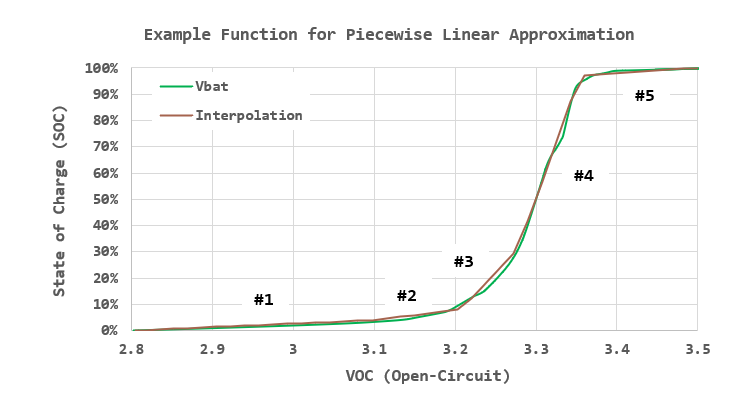# Optimized Piecewise Linear Model Using Excel

Quote of the Day

Never respond too quickly or too well to a request that you do not want to see more of in the future.

— A truism of corporate life. This is my paraphrase of a statement by a coworker, Nick Priote, who often made very insightful observations on the corporate world vs academia.

## Introduction

I was recently asked to create a piecewise linear model for a rather complex battery discharge curve, which is a type of task that I have performed dozens of times. I was told to perform this task in Excel because that is the only computation tool that this customer uses. I normally do this task in R because I like the segmented package, however, Excel does a very good job with the task, especially if you use the Solver add-in to "tune" the model.

Figure 1 is the kind of curve I normally get from our test battery folks, which is the open-circuit battery voltage (VOC) vs State of Charge (SOC). The software folks, my customers for this modeling effort, typically know VOC and want to know SOC, so they care about the SOC vs VOC curve. This requires that you flip the abscissa and ordinate values, which is a minor transformation.

Because the actual battery data is proprietary, I will create a piecewise-linear approximation to a discharge curve I found on the web (Figure 1). For those who like to follow along, my workbook is available here.

## Background

### Definitions

Breakpoint (Knee Point)
The point on the curve where the approximation transitions from one line segment to another. Because we need continuity, the line segments must have the same value at the breakpoint.
Objective Function
This is the error measure that our algorithm will be minimizing.
Solver
Solver is an add-in for Excel. This is an unbelievably powerful tool that many folks do not even know exists. The Solver that is included in Excel is a reduced functionality version of a more extensive package from Frontline Systems. There is also an open-source version (link) under development.

### Optimization

There are a number of different optimization criteria we could apply to this problem, but for this exercise, I will be minimizing the maximum absolute error. You could easily change the algorithm to minimize the sum of the least square errors or a weighted error approach.

In the real battery problem (not shown in the workbook), I actually weighted the battery capacity errors more heavily when the battery state of charge was low versus when the state of charge was high. This is because people generally want more accuracy when their battery is nearly out than when it is full. I have done similar optimizations for aircraft fuel systems where customers are less concerned with accuracy when the tanks are full than when they are near empty. This is yet another manifestation of the utility curve from economics – stuff has increased value when you are short of it.

## Analysis

### Setup

For this example, I am going to use five line segments to approximate a cubic function. For the actual problem that I worked, my measured battery curve looked nothing like a cubic function, but the process for determining the piecewise linear approximation is the same.

Here is the process I used (there are many possible alternatives):

• Assume the State Of Charge (SOC) and battery voltages are known at the end points of battery voltage range: (VOC=2.8 V, SOC=0%), (VOC=3.5 V, SOC = 100%).
• Pick three points (voltages) in the middle of the VOC range (2.8 V to 3.5 V).
• Determine lines between each of these points
• Setup a data table with a large number of points that encompass the whole VOC range.
• Use the data table to compute a SOC value for every VOC point.
• Determine the error between the model SOC value and measured SOC value (some interpolation will be needed for the measured SOC values).
• Compute the maximum absolute error of the absolute differences between the model SOC values and measured SOC values.
• Use Solver to select (VOC, SOC) values to minimize the absolute errors. The initial guess must be fairly close to the final values to ensure a good approximation.

### Optimization

See the workbook to see how the optimization is setup. One issue with this approach is that your starting values must be good for Solver to converge to a good solution.

### Result

Figure 2 shows the battery curve of Figure 1 and my interpolated results. Note that the curve of Figure 1 shows VOC vs SOC and Figure 2 shows SOC vs VOC, which is what the firmware folks I work with need. All I needed to do was flip the abscissa and ordinate values.## Conclusion

Generating piecewise linear approximations for non-linear curves is a very common task. In my career, about 90% of this work has involved batteries (always nonlinear) or sensors (frequently nonlinear).

One frequent problem is that the SOC vs VOC curve is frequently not a one-to-one function. This means that the VOC does not map to a unique SOC value. This makes SOC determination much more difficult, which means it will be a topic for another post.

This entry was posted in Batteries, Excel. Bookmark the permalink.